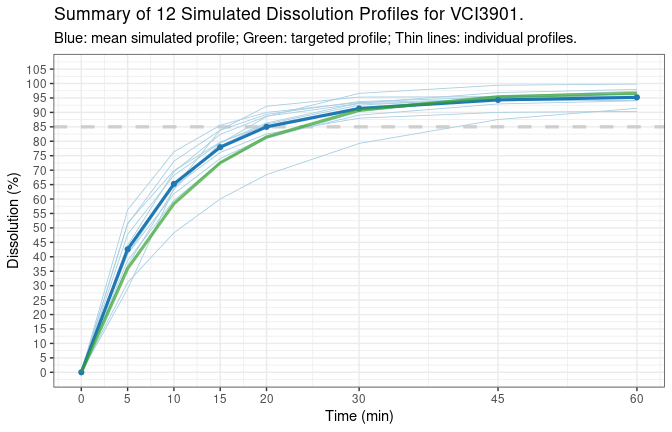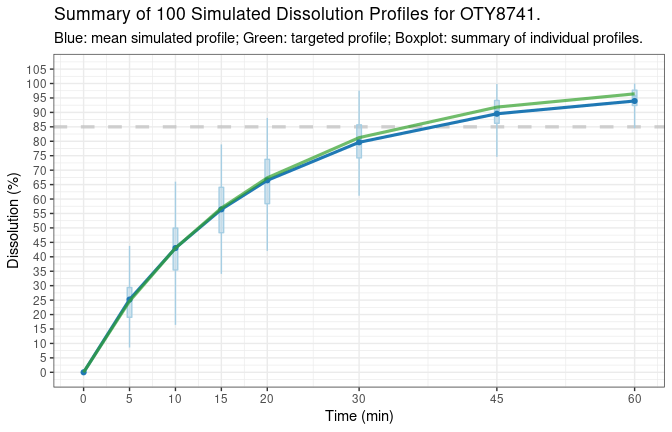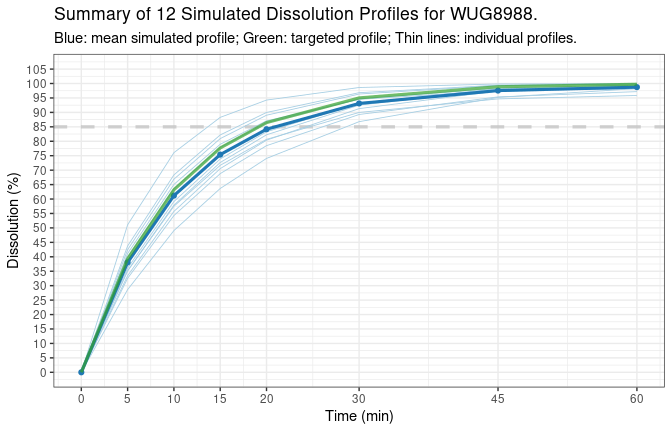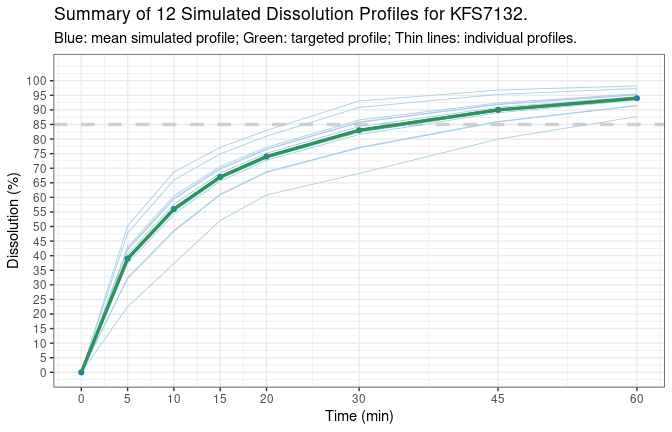# Simulation of Dissolution Profiles

## Introduction

Dissolution profiles are simulated either by mathematical models, or by multivariate normal distribution based on function mvrnorm() from R package MASS.

### Mathematical Models

Two models are implemented at the moment: first-order model and Weibull model (the default).

The first-order model is expressed as

$f_t = f_\mathrm{max}\left(1 - e^{-k\cdot (t - t_\mathrm{lag})}\right),$ and the Weibull model either as $f_t = f_\mathrm{max}\left(1 - e^{-\left(\frac{t - t_\mathrm{lag}} {\mathrm{MDT}}\right)^\beta}\right),$ or as $f_t = f_\mathrm{max}\left(1 - e^{-\frac{\left(t - t_\mathrm{lag}\right)^\beta} {\alpha}}\right).$ Obviously, the two expressions of Weibull model are mathematically equivalent with $$\alpha = \mathrm{MDT}^\beta$$.

Parameter $$f_\mathrm{max}$$ is the maximum dissolution. In theory, it is 100, but in practice, it might be slightly high than 100, or much less if the dissolution is not complete. For immediate-release formulation, the lag time $$t_\mathrm{lag}$$ is typically zero, but not necessary so for the extended release formulation. Parameter $$k$$ is the first-order rate constant, and $$\alpha$$ and $$\beta$$ are scale and shape parameters, and $$\mathrm{MDT}$$ is the mean dissolution time.

### Simulation based on mathematical models

Let $$P_\mu$$ be the set of population model parameters, i.e., $$P_\mu = \{f_\mathrm{max}, k, t_\mathrm{lag}\}$$ for the first-order model and $$P_\mu = \{f_\mathrm{max}, t_\mathrm{lag}, \mathrm{MDT}, \beta\}$$ or $$P_\mu = \{f_\mathrm{max}, t_\mathrm{lag}, \alpha, \beta\}$$ for Weibull model, given any time points tp, the dissolution profile $$f_t$$ will be calculated according to the equations above with parameters $$P_\mu$$. The generated profile is considered as population dissolution profile dp.

Individual dissolution profiles are generated with the same equation, but with individual model parameters $$P_i$$, which is simulated according to exponential error model $P_i = P_\mu \cdot e^{N\left(0,\, \sigma^2\right)},$ where $$N\left(0,\, \sigma^2\right)$$ represents the normal distribution with mean zero and variance $$\sigma^2$$ ($$\sigma = \mathrm{CV}/100$$)

### Simulation based on multivariate normal distribution

Note that the population dissolution profile dp in the previous section is generated by equation with population model parameters $$P_\mu$$. Sometime we might have a mean dissolution profile dp, which is considered as population profile, and we want to simulate many individual profiles such that the calculated mean profile is equal to dp. In such case, multivariate normal distribution approach will be used. Given any tp, dp, and the required number of individual units, n.units, the function will simulate individual profiles fulfil the above mentioned requirements.

### Recommendation

Depending on the variability and the target profile, the run time of simulation based on multivariate normal distribution might be very long, sometimes it might even fail to finish. So in general, the modelling approach is recommended.

## Usage

The complete list of arguments of the function is as follows:

sim.dp(tp, dp, dp.cv, model = c("Weibull", "first-order"),
model.par = NULL, seed = NULL, n.units = 12L, product,
max.disso = 100, ascending = FALSE, message = FALSE,
time.unit = c("min", "h"), plot = TRUE,
plot.max.unit = 36L)

The approach used to simulate individual dissolution profiles depends on if the target mean dissolution profile dp is provided by the user or not.

• If dp is not provided, then it will be calculated using tp, model, and model.par. The parameters defined by model.par are considered as the population parameter; consequently, the calculated dp is considered as the targeted population profile. In addition, n.units sets of individual model parameters will be simulated based on exponential error model, and individual dissolution profiles will be generated using those individual parameters. The mean of all simulated individual profiles, sim.mean, included in one of the outputs data frames, sim.summary, will be similar, but not identical, to dp. The difference between sim.mean and dp is determined by the number of units and the variability of the model parameters. In general, the larger the number of units and the lower of the variability, the smaller the difference.
• If dp is provided, then n.units of individual dissolution profiles will be simulated using multivariate normal distribution. The mean of all simulated individual profiles, sim.mean, will be identical to dp. In such case, it is recommended that dp.cv, the CV at time points tp, should also be provided by the user. If dp.cv is not provided, it will be generated automatically such that the CV is between 10% and 20% for time points up to 10 min, between 1% and 3% for time points where the dissolution is more than 95%, between 3% and 5% for time points where the dissolution is between 90% and 95%, and between 5% and 10% for the rest of time points. Whether the dp.cv is provided or generated automatically, the CV calculated using all individual profiles will be equal to dp.cv.

### Minimum required arguments that must be provided by the user

If dp is provided by the user, logically, tp must also be provided, and the approach based on multivariate normal distribution is used, as explained above. If dp is not provided, tp could be missing, i.e., a simple function call such as sim.dp() will simulate dissolution profiles. In such case, a default tp will be generated depending on the time.unit: if the time.unit is "min", then tp would be c(5, 10, 15, 20, 30, 45, 60); otherwise the tp would be c(1, 2, 3, 4, 6, 8, 10, 12). The rest arguments are either optional or required by the function but default values will be used.

### Notes on function arguments

1. Model parameter model.par has to be specified as named list, e.g., list(fmax = 100, fmax.cv = 3, tlag = 0, tlag.cv = 0, k = 0.9, k.cv = 30). The parameter fmax/k/tlag are used to generate dp, and the corresponding .cv parts are used to generate individual model parameters. If model.par is missing, it will be generated randomly.
2. When multivariate normal distribution approach is used, depending on the target profile and the variability, sometimes the simulated individual profiles will decrease in the end. This could also happen to the real-life profiles, especially for those products that are unstable in the dissolution media. To force the profiles always increase with time, set option ascending = TRUE. However, in that case, it is possible that the function takes long time to run or even fails.
3. Parameter product is not really necessary for the simulation. so if missing, it will be generated automatically with 3 letters and 4 digits. It might be useful in the situations such as many simulation will be run and output are pooled together for analysis.
4. Read the function manual by help("sim.dp") for more details on each argument.

## Examples

### Simple case

For the most basic use, the function can be run without any user provided arguments, e.g., sim.dp(). In such case, 12 units of individual dissolution profiles will be simulated using Weibull model with a typical sampling time points of 5, 10, 15, 20, 30, 45, and 60 min. A seed number will be randomly generated, if not provided by the user, and included in the output for reproducibility purpose.

# simulation
tmp1 <- sim.dp(seed = 1234)

The output is a list of 5 components:

1. sim.summary: A data frame with summary statistics of all individual dissolution profiles.
tmp1$sim.summary # product time dp dp.cv sim.mean sim.median sim.cv sim.var sim.sd # 1 VCI3901 0 0.00000 NA 0.00000 0.00000 0.000000 0.000000 0.000000 # 2 VCI3901 5 35.91205 NA 42.59002 41.74175 19.312496 67.653855 8.225196 # 3 VCI3901 10 58.37878 NA 65.24386 64.63399 11.048803 51.964863 7.208666 # 4 VCI3901 15 72.55031 NA 77.95186 79.42119 8.856818 47.665970 6.904055 # 5 VCI3901 20 81.51082 NA 85.00156 86.16105 7.383728 39.391744 6.276284 # 6 VCI3901 30 90.78021 NA 91.34221 92.78433 4.956413 20.496418 4.527297 # 7 VCI3901 45 95.42728 NA 94.32405 95.06778 3.258574 9.447131 3.073618 # 8 VCI3901 60 96.61781 NA 95.21829 95.38719 2.752588 6.869471 2.620968 # sim.min sim.max sim.qt05 sim.qt25 sim.qt75 sim.qt95 # 1 0.00000 0.00000 0.00000 0.00000 0.00000 0.00000 # 2 28.86682 56.23244 30.08120 38.28761 48.65758 53.69296 # 3 48.29650 76.37401 54.49829 62.94728 69.54749 74.58668 # 4 60.03121 85.53969 66.92377 75.59784 82.76237 84.65364 # 5 68.43588 92.13902 75.32032 82.27256 88.85582 90.96216 # 6 79.25227 96.60286 84.06618 89.90644 93.51285 95.88751 # 7 87.54060 99.40223 88.90028 93.78673 95.48357 98.00911 # 8 90.36151 99.80318 90.94327 94.12407 96.65417 98.79344 dp is the population mean profile obtained with the parameters in sim.info, as explained in previous section. The rest columns with prefix sim are basic descriptive statistics calculated from all simulated individual profiles. sim.qt05, sim.qt25, …, are 5%, 25%, .., quantiles. 1. sim.disso: A data frame with all individual dissolution profiles. tmp1$sim.disso
#   time  unit.01  unit.02  unit.03  unit.04  unit.05  unit.06  unit.07  unit.08
# 1    0  0.00000  0.00000  0.00000  0.00000  0.00000  0.00000  0.00000  0.00000
# 2    5 42.44302 38.68705 47.76202 31.07478 28.86682 41.04047 40.98547 51.34429
# 3   10 64.34095 59.57248 68.16946 48.29650 63.68414 64.92703 61.81370 73.12431
# 4   15 76.12156 72.56313 79.54749 60.03121 83.92869 78.42522 74.02670 83.83484
# 5   20 82.53928 80.95304 86.33855 68.43588 92.13902 85.98356 81.47238 89.33110
# 6   30 88.00484 90.19166 93.22817 79.25227 95.30223 92.52769 89.05079 93.77081
# 7   45 90.01275 95.58009 96.86928 87.54060 95.45140 94.92385 92.93529 95.32524
# 8   60 90.36151 97.34724 97.96729 91.41926 95.45178 95.32260 94.01854 95.59138
#    unit.09  unit.10  unit.11  unit.12
# 1  0.00000  0.00000  0.00000  0.00000
# 2 43.93941 56.23244 51.61520 37.08931
# 3 69.44566 76.37401 69.85299 63.32513
# 4 82.40489 85.53969 79.70409 79.29489
# 5 88.69740 89.99927 85.58302 88.54620
# 6 93.04097 93.42686 91.70737 96.60286
# 7 94.07055 94.56556 95.21171 99.40223
# 8 94.15925 94.75433 96.42314 99.80318
1. sim.info: A data frame with information of the simulation.
tmp1$sim.info # fmax fmax.cv mdt mdt.cv beta beta.cv tlag tlag.cv seed n.units # 1 97.03 3 10.87 30 0.993764 20 0 0 1234 12 # max.disso model time.unit # 1 100 Weibull min 1. model.par.ind: A data frame of individual model parameters that are used to simulate the individual dissolution profiles if mathematical models are chosen for the simulation. tmp1$model.par.ind
#    fmax.ind   mdt.ind  beta.ind tlag.ind
# 1  90.43662  7.995749 0.9720844        0
# 2  98.28721 10.820746 0.8972171        0
# 3  98.51432  8.208926 0.8282050        0
# 4  95.37133 15.130249 0.8405575        0
# 5  95.45179  9.424652 1.6110909        0
# 6  95.40077  8.786133 1.0207750        0
# 7  94.47347  9.352365 0.9008715        0
# 8  95.65084  6.667725 0.9099505        0
# 9  94.16689  7.657771 1.0894384        0
# 10 94.79651  5.651917 0.8650227        0
# 11 97.21781  7.269654 0.7438644        0
# 12 99.86358  9.951453 1.1148230        0
1. sim.plot: A plot since the default argument plot is set to be TRUE.
tmp1$sim.plotWhen the number of individual units is not large, all individual profiles will be plotted, together with the population profile in green (as target profile), and mean profile in blue calculated with simulated individual profiles. When the number is small, there will be visible difference between the mean and the target profile due to random error. When the number of units increase, the difference will become smaller. The argument plot.max.unit control how individual profile will be represented in the plot. When the actual number of units is greater than the value of plot.max.unit, the individual profile will be represented as boxplots at each time points, as shown below. # default plot.max.unit = 36 sim.dp(n.units = 100)$sim.plot### Example with model parameters

To obtain better controlled simulation, model parameters should be provided. CV should be specified in percentage.

fo.par <- list(fmax = 100, fmax.cv = 3, k = 0.1, k.cv = 20,
tlag = 0, tlag.cv = 0)
fo.dat <- sim.dp(model = "first-order", model.par = fo.par, seed = 123)
fo.dat$sim.plotfo.dat$sim.summary
#   product time       dp dp.cv sim.mean sim.median    sim.cv   sim.var   sim.sd
# 1 WUG8988    0  0.00000    NA  0.00000    0.00000  0.000000  0.000000 0.000000
# 2 WUG8988    5 39.34693    NA 38.05432   36.80249 15.742046 35.886428 5.990528
# 3 WUG8988   10 63.21206    NA 61.19125   59.96058 11.854418 52.618582 7.253867
# 4 WUG8988   15 77.68698    NA 75.38813   74.53645  8.860120 44.615441 6.679479
# 5 WUG8988   20 86.46647    NA 84.17413   83.71286  6.589706 30.767292 5.546827
# 6 WUG8988   30 95.02129    NA 93.09927   93.13109  3.653316 11.568235 3.401211
# 7 WUG8988   45 98.88910    NA 97.55660   97.76842  1.739297  2.879127 1.696799
# 8 WUG8988   60 99.75212    NA 98.74321   99.17107  1.259422  1.546526 1.243594
#    sim.min  sim.max sim.qt05 sim.qt25 sim.qt75 sim.qt95
# 1  0.00000  0.00000  0.00000  0.00000  0.00000  0.00000
# 2 28.63804 51.07058 30.78849 34.68072 41.45634 47.04206
# 3 49.07471 76.05912 51.93852 57.06366 65.50805 71.82178
# 4 63.65871 88.28587 66.48323 71.39243 79.46243 84.93773
# 5 74.06614 94.26834 76.49645 80.59899 87.55868 91.91297
# 6 86.79311 98.62779 88.15907 90.98362 95.10721 97.63956
# 7 94.67076 99.83926 94.96209 96.85160 98.56235 99.61739
# 8 95.86586 99.98117 96.59454 98.23806 99.61743 99.93629

Similarly, for Weibull model, the model parameter should be provided like the example below. The order of the parameter does not matter, but the parameter names have to be specified exactly as the following:

# with alpha = xx and alpha.cv = yy to replace beta/beta.cv if alternative
# expression of Weibull model is used.
mod.par <- list(fmax = 100, fmax.cv = 3, tlag = 0, tlag.cv = 0,
mdt = 20, mdt.cv = 25, beta = 2, beta.cv = 30)

### Example with multivariate normal distribution

# target mean profile
dp <- c(39, 56, 67, 74, 83, 90, 94)

# CV at each time points
dp.cv <- c(19, 15, 10, 8, 8, 5, 3)

mvn.dat <- sim.dp(tp, dp = dp, dp.cv = dp.cv, seed = 1234)
mvn.dat$sim.summary # product time dp dp.cv sim.mean sim.median sim.cv sim.var sim.sd sim.min # 1 KFS7132 0 0 0 0 0.00000 0 0.0000 0.00 0.00000 # 2 KFS7132 5 39 19 39 39.96268 19 54.9081 7.41 22.50088 # 3 KFS7132 10 56 15 56 57.09130 15 70.5600 8.40 37.29655 # 4 KFS7132 15 67 10 67 67.87044 10 44.8900 6.70 52.08177 # 5 KFS7132 20 74 8 74 74.76911 8 35.0464 5.92 60.81852 # 6 KFS7132 30 83 8 83 83.86265 8 44.0896 6.64 68.21537 # 7 KFS7132 45 90 5 90 90.58463 5 20.2500 4.50 79.98029 # 8 KFS7132 60 94 3 94 94.36637 3 7.9524 2.82 87.72098 # sim.max sim.qt05 sim.qt25 sim.qt75 sim.qt95 # 1 0.00000 0.00000 0.00000 0.00000 0.00000 # 2 50.21427 27.84993 36.17045 42.41837 48.87195 # 3 68.71253 43.36024 52.79241 59.87508 67.19087 # 4 77.13976 56.91829 64.44157 70.09084 75.92605 # 5 82.95931 65.09198 71.73941 76.73101 81.88690 # 6 93.04896 73.00857 80.46448 86.06316 91.84612 # 7 96.81029 83.22870 88.28165 92.07593 95.99511 # 8 98.26778 89.75665 92.92317 95.30092 97.75693 Notice that the the mean and CV of the simulated individual profile ( sim.mean and sim.cv) are equal to the target profile dp and dp.cv. The plot look like this: mvn.dat$sim.plotAnother example with missing dp.cv.

mvn.dat2 <- sim.dp(tp, dp = dp, seed = 123)
mvn.dat2\$sim.summary
#   product time dp dp.cv sim.mean sim.median sim.cv sim.var sim.sd  sim.min
# 1 JFG9080    0  0     0        0    0.00000      0  0.0000   0.00  0.00000
# 2 JFG9080    5 39    12       39   39.12472     12 21.9024   4.68 31.86723
# 3 JFG9080   10 56    12       56   56.17909     12 45.1584   6.72 45.75808
# 4 JFG9080   15 67     7       67   67.12499      7 21.9961   4.69 59.85199
# 5 JFG9080   20 74     7       74   74.13805      7 26.8324   5.18 66.10518
# 6 JFG9080   30 83     7       83   83.15484      7 33.7561   5.81 74.14500
# 7 JFG9080   45 90     4       90   90.09594      4 12.9600   3.60 84.51326
# 8 JFG9080   60 94     4       94   94.10021      4 14.1376   3.76 88.26940
#    sim.max sim.qt05 sim.qt25 sim.qt75 sim.qt95
# 1  0.00000  0.00000  0.00000  0.00000  0.00000
# 2 45.81756 32.10244 36.25681 43.06469 45.46386
# 3 65.78932 46.09581 52.06105 61.83647 65.28145
# 4 73.83213 60.08770 64.25094 71.07337 73.47768
# 5 81.54593 66.36552 70.96373 78.49895 81.15445
# 6 91.46368 74.43700 79.59445 88.04612 91.02458
# 7 95.24428 84.69418 87.88985 93.12668 94.97220
# 8 99.47736 88.45837 91.79607 97.26565 99.19319

Notice that the dp.cv were generated automatically as explained in Notes on function arguments.